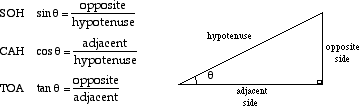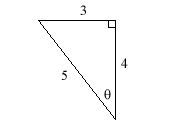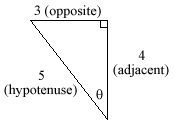# sohcahtoa

SOHCAHTOA

A way of remembering how to compute the sine, cosine, and tangent of an angle.

SOH stands for Sine equals Opposite over Hypotenuse.

CAH stands for Cosine equals Adjacent over Hypotenuse.

TOA stands for Tangent equals Opposite over Adjacent.Example: Find the values of sin θ,cos θ, and tan θ in the right triangle shown.Answer: sin θ = 3/5 = 0.6cosθ = 4/5 = 0.8tanθ = 3/4 = 0.75This triangle is oriented differently than the one shown in the SOHCAHTOA diagram, so make sure you know which sides are the opposite, adjacent, and hypotenuse.